1. Home
2. >
3. Blog
4. >

# Deviations from Beer-Lambert law

by egpat         05 Jun 2019

Relation between absorbance and concentration of an analyte is given by Beer-Lambert law. Is that law valid in any condition? Does it have any limitation? Yes, of course, as with any theoretical law there may be a practical limitation. Here we will discuss such limitation of Beer-Lambert law and where it is applied perfectly.

## Deviation from linearity

One of the simplest advantages of Beer-Lambert law is its linearity of absorbance with concentration. For an analyte when temperature, path length and wavelength of radiation are kept constant, the absorbance is directly proportional to concentration.

A=abc

In another words, the absorbance of a sample will be doubled when concentration is doubled maintaining the linear proportionality between them.

So when we plot a graph between absorbance and concentration we should get a straight line passing through origin.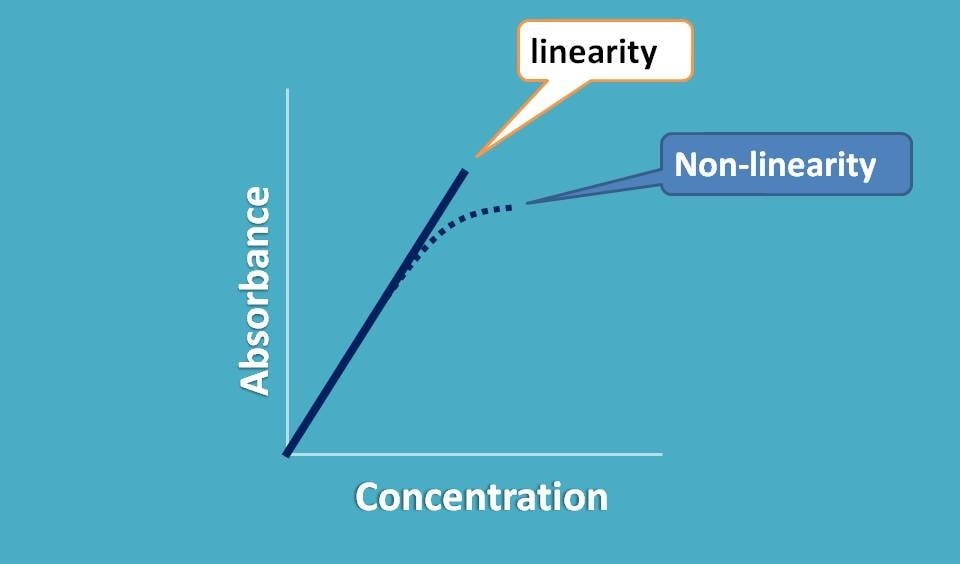Now if it doesn’t behave like this, what happens? We observe a deviation from linearity and thereby deviation in Beer-Lambert law.

This non-linearity can be produced by variety of the situations which we can categorize mainly into three categories.

• Real deviations
• Spectral deviations
• Chemical deviations

We will go with one by one in detail.

## Real deviation

This deviation is due to the analyte itself particularly observed at higher concentrations of analyte.

Which concentration you may use for detecting absorbance in spectrophotometer?

Suppose you have placed a concentrated solution and recorded the absorbance as A1. Now you have diluted the sample to half of its concentration and again recorded the absorbance as A2. What you can expect? Since you have diluted the concentration to the half, the absorbance should also be reduced to half. In another words A2=A­1/2.But in reality, it may not happen like that and A2 may not be equal to A1/2. That’s why it is called real deviation.

What makes the non-linearity here?

Here we should think not only of concentration but also how it affects the absorption process. For example, the speed of light passing in the higher concentration may not be equal to that in lower concentration.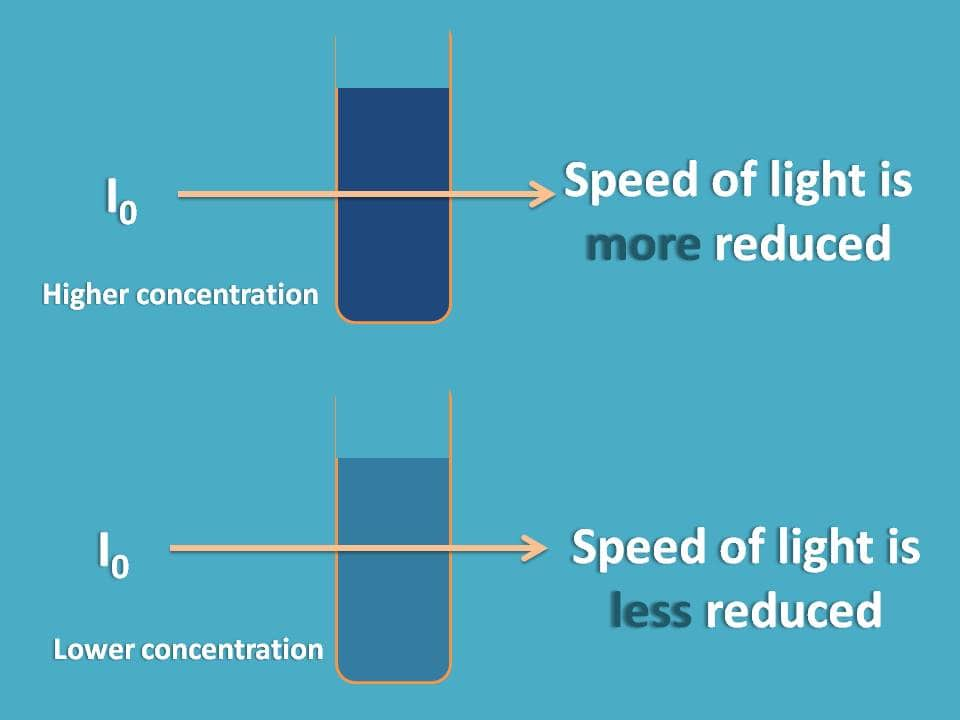We know that speed of the light may vary with the medium though which it passes. This can be given by refractive index which is the ration of velocities of light in the medium to vacuum. For practical purpose, with dilute solutions refractive index becomes nearer to 1.So in the lower concentrations, refractive index is nearer to 1 but at higher concentrations it is less than 1 and affects the speed of light significantly bringing a deviation from Beer-Lambert law.

Beer-Lambert law assumes that the refractive indices of all the samples measured is same and ideally nearer to 1.

Then how can we eliminate this real deviation?

Simply it can be eliminated by dilution. Yes, when deviation occurs at higher concentrations, why should we go for such concentrations, let’s dilute all the samples such that refractive index is same and equal to 1 in all the samples.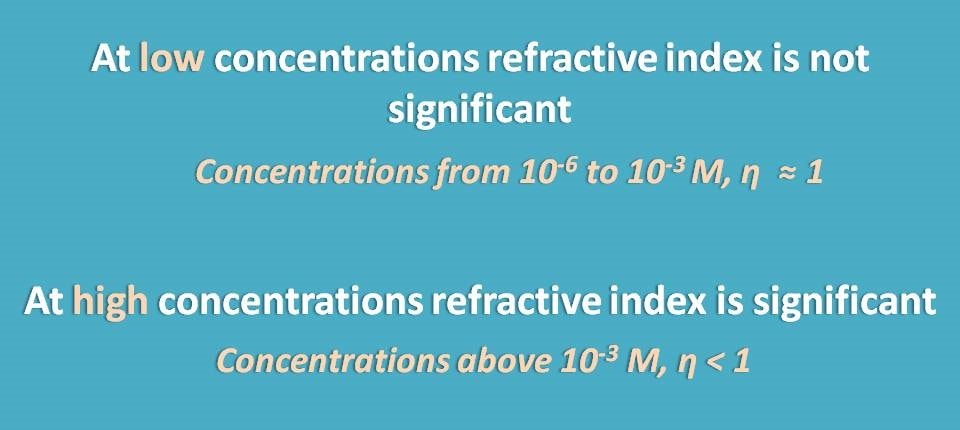That’s why we normally use the concentration of the sample at micromolar(μM) level.  For example, we can prepare a series of concentrations o f the sample at 0,5μM, 10μM, 20μM, 40μM etc.

## Spectral deviation

It is another deviation that mainly related to the instrument. Which type of radiation we use for measuring absorbance. Undoubtedly, it is monochromatic radiation with a narrow range of wavelengths. If we irradiate the sample with polychromatic radiation, simply deviation from Beer-Lambert law is produced.

Consider an example. Suppose a sample shows maximum absorption at 560 nm and you are sending a radiation of wavelengths from 550 to 570 nm. As the band width of radiation is only 20 nm , it can obey Beer-Lambert law.On the other hand, if you send a radiation of wavelength range 520 nm to 600 nm, you may not achieve linearity and deviation occurs.

So when you use polychromatic radiation, Beer-Lambert law is not strictly obeyed and linearity is not observed.

Again how can we eliminate this spectral deviation?

As this deviation is with polychromatic radiation, you have to select a suitable monochromator which allows only a narrow range of wavelengths of radiation to pass through the sample.The range of wavelengths passed by monochromator depends on its slit width. As a general approach, we should select a monochromator with silt width as at least one tenth of the natural bandwidth of the analyte.

Suppose an analyte absorbs from 460 to 660 nm with λmax at 560 nm, its natural band with is equal to 660-460=200 nm.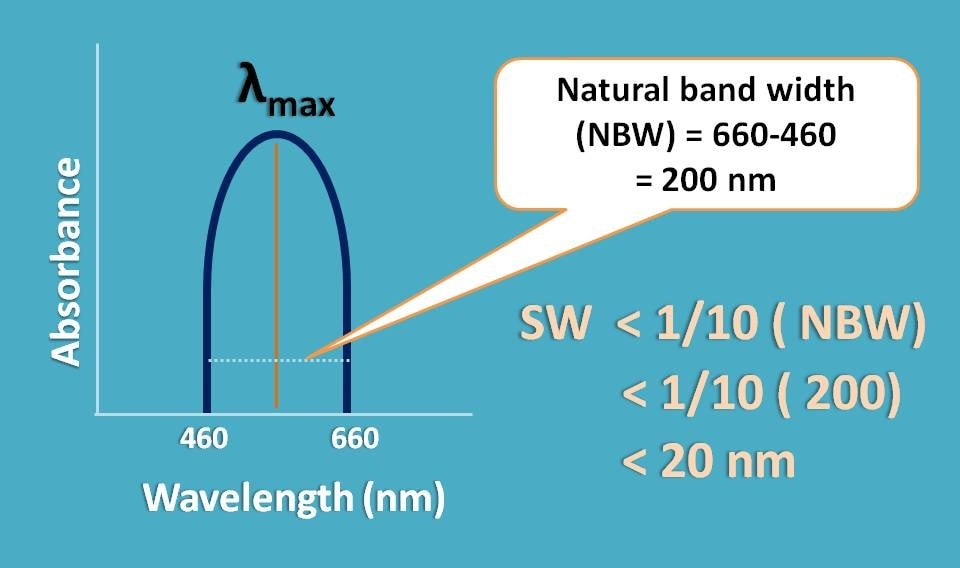In that case we have to select a monochromator with slit width less than one-tenth of natural band width, that is, 1/10(200)=20 nm.

## Chemical deviation

Finally, another type of deviation may occur due to any chemical changes in the analyte throughout the samples. These may include association, dissociation or solvent interactions.

Analyte at low concentration may be inert but at high concentration it may undergo association or even dissociation. Interaction with solvent molecules may also be observed at higher concentrations which cause non-linearity at the end of the plot.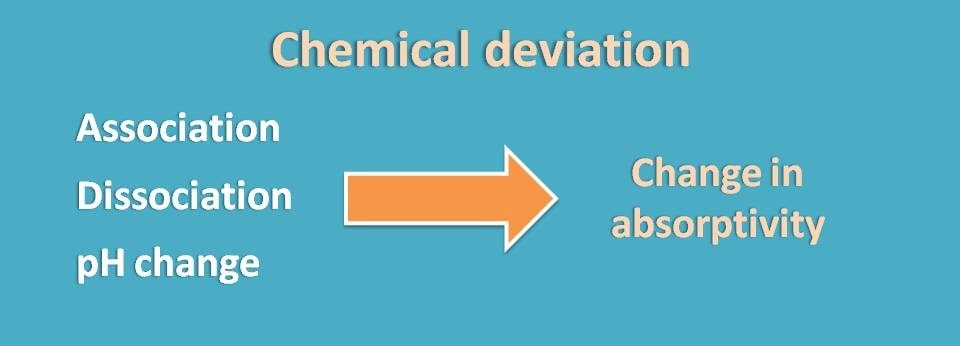Sometimes a change in the pH can also bring changes in the absorbance. So it is better to fix the pH by using a suitable buffer whenever required.